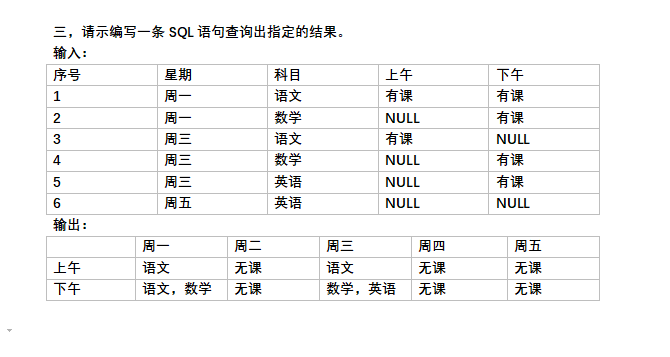•闪存
•博客
• 发言小组
• 投递新闻
• 提问博问
• 添加收藏
•文库

# 一道SQL面试题

0悬赏园豆：50 [已解决问题] 解决于 2018-11-08 11:530

select 日期, IFNULL(Max(case m.周期 when '周一' then 科目 end),"无课") as '周一' ,
IFNULL(Max(case m.周期 when '周二' then 科目 end),"无课") as '周二' ,
IFNULL(Max(case m.周期 when '周三' then 科目 end),"无课") as '周三',
IFNULL(Max(case m.周期 when '周四' then 科目 end) ,"无课")as '周四',
IFNULL(Max(case m.周期 when '周五' then 科目 end),"无课") as '周五' from (
select 周期,GROUP_CONCAT(科目) as 科目,'上午' as 日期 from `课表` where 上午='有课' GROUP BY 周期 ) m GROUP BY 日期
UNION
select 日期, IFNULL( Max(case m.周期 when '周一' then 科目 end),"无课")as '周一' ,
IFNULL(Max(case m.周期 when '周二' then 科目 end),"无课") as '周二' ,
IFNULL(Max(case m.周期 when '周三' then 科目 end),"无课") as '周三',
IFNULL(Max(case m.周期 when '周四' then 科目 end),"无课") as '周四',
IFNULL(Max(case m.周期 when '周五' then 科目 end),"无课") as '周五' from (
select 周期,GROUP_CONCAT(科目) as 科目,'下午' as 日期 from `课表` where 下午='有课' GROUP BY 周期 ) m GROUP BY 日期　　　　　　　　　　　　　　　　　　　　　　　　　　　　　　　　　　　　　　　　　　　　　　　　　　　　　　　　　　　　　　　　　　　　　　　　　　　　　　　　　　　　　　　　　　　　　　　　　　　　　　　　　　　　如果有更简单的写法，请大佬指正～～～

select '上午' AS " ",
max(case when 星期 = '周一' AND 上午='有课' then (select GROUP_CONCAT(科目) FROM a WHERE 上午='有课' AND 星期='周一') ELSE '无课' end) 周一,
max(case when 星期 = '周二' AND 上午='有课' then (select GROUP_CONCAT(科目) FROM a WHERE 上午='有课' AND 星期='周二') ELSE '无课' end) 周二,
max(case when 星期 = '周三' AND 上午='有课' then (select GROUP_CONCAT(科目) FROM a WHERE 上午='有课' AND 星期='周三') ELSE '无课' end) 周三,
max(case when 星期 = '周四' AND 上午='有课' then (select GROUP_CONCAT(科目) FROM a WHERE 上午='有课' AND 星期='周四') ELSE '无课' end) 周四,
max(case when 星期 = '周五' AND 上午='有课' then (select GROUP_CONCAT(科目) FROM a WHERE 上午='有课' AND 星期='周五') ELSE '无课' end) 周五
from a
union
select '下午' AS " ",
max(case when 星期 = '周一' AND 下午='有课' then (select GROUP_CONCAT(科目) FROM a WHERE 下午='有课' AND 星期='周一') ELSE '无课' end) 周一,
max(case when 星期 = '周二' AND 下午='有课' then (select GROUP_CONCAT(科目) FROM a WHERE 下午='有课' AND 星期='周二') ELSE '无课' end) 周二,
max(case when 星期 = '周三' AND 下午='有课' then (select GROUP_CONCAT(科目) FROM a WHERE 下午='有课' AND 星期='周三') ELSE '无课' end) 周三,
max(case when 星期 = '周四' AND 下午='有课' then (select GROUP_CONCAT(科目) FROM a WHERE 下午='有课' AND 星期='周四') ELSE '无课' end) 周四,
max(case when 星期 = '周五' AND 下午='有课' then (select GROUP_CONCAT(科目) FROM a WHERE 下午='有课' AND 星期='周五') ELSE '无课' end) 周五
from a

@路边一草鞋: 你没有聚合，去取max 最大值的时候取到了第一个，，第一条数据是无课，所以地周三的下午是无课，周一有数据是因为，第一条就是 课程信息，，select '下午' AS " ",
case when 周期 = '周一' AND 下午='有课' then (select GROUP_CONCAT(科目) FROM 课表 WHERE 下午='有课' AND 周期='周一') ELSE '无课' end 周一
from 课表 用上面这个语句执行一下，然后换成周三，在执行一次你就明白了，我那个语句也是mysql 写的

select 日期,
IFNULL(Max(case m.星期 when '周一' then 科目 end),"无课") as '周一' ,
IFNULL(Max(case m.星期 when '周二' then 科目 end),"无课") as '周二' ,
IFNULL(Max(case m.星期 when '周三' then 科目 end),"无课") as '周三',
IFNULL(Max(case m.星期 when '周四' then 科目 end) ,"无课")as '周四',
IFNULL(Max(case m.星期 when '周五' then 科目 end),"无课") as '周五'
from
(select 星期,GROUP_CONCAT(科目) as 科目,'上午' as 日期 from a where 上午='有课' GROUP BY 星期 ) m
GROUP BY 日期
UNION
select 日期,
IFNULL(Max(case m.星期 when '周一' then 科目 end),"无课") as '周一' ,
IFNULL(Max(case m.星期 when '周二' then 科目 end),"无课") as '周二' ,
IFNULL(Max(case m.星期 when '周三' then 科目 end),"无课") as '周三',
IFNULL(Max(case m.星期 when '周四' then 科目 end),"无课") as '周四',
IFNULL(Max(case m.星期 when '周五' then 科目 end),"无课") as '周五'
from
(select 星期,GROUP_CONCAT(科目) as 科目,'下午' as 日期 from a where 下午='有课' GROUP BY 星期 ) m
GROUP BY 日期

1

https://www.cnblogs.com/WangShuaishuai/p/9407917.html

0

fangq | 园豆：417 (菜鸟二级) | 2018-11-06 15:35
0

MYSQL的
select
a.时间,ifnull(group_concat(a.周一), '无课') 周一,
ifnull(group_concat(a.周二), '无课') 周二,
ifnull(group_concat(a.周三), '无课') 周三,
ifnull(group_concat(a.周四), '无课') 周四,
ifnull(group_concat(a.周五), '无课') 周五
from
(
SELECT
'上午' as 时间,
group_concat( case week_dat when '周一' then class else null end) AS 周一,
group_concat( case week_dat when '周二' then class else null end) AS 周二,
group_concat( case week_dat when '周三' then class else null end) AS 周三,
group_concat( case week_dat when '周四' then class else null end) AS 周四,
group_concat( case week_dat when '周五' then class else null end) AS 周五
from class_detail
where morning is not null
GROUP BY week_dat
union all

SELECT
'下午' as 时间,
group_concat( case week_dat when '周一' then class else null end) AS 周一,
group_concat( case week_dat when '周二' then class else null end) AS 周二,
group_concat( case week_dat when '周三' then class else null end) AS 周三,
group_concat( case week_dat when '周四' then class else null end) AS 周四,
group_concat( case week_dat when '周五' then class else null end) AS 周五
from class_detail
where afternoon is not null
GROUP BY week_dat
) a
group by 时间

您需要登录以后才能回答，未注册用户请先注册